•#### IPython Notebook Essentials#### Overview of this book

IPython Notebook EssentialsCreditswww.PacktPub.comPrefaceFree Chapter
A Tour of the IPython NotebookThe Notebook InterfaceGraphics with matplotlibHandling Data with pandasAdvanced Computing with SciPy, Numba, and NumbaProIPython Notebook Reference CardA Brief Review of PythonNumPy ArraysIndex## Example – the coffee cooling problem

Suppose you get a cup of coffee at a coffee shop. Should you mix the cream into the coffee at the shop or wait until you reach your office? The goal, we assume, is to have the coffee as hot as possible. So, the main question is how the coffee is going to cool as you walk back to the office.

The difference between the two strategies of mixing cream is:

• If you pour the cream at the shop, there is a sudden drop of temperature before the coffee starts to cool down as you walk back to the office

• If you pour the cream after getting back to the office, the sudden drop occurs after the cooling period during the walk

We need a model for the cooling process. The simplest such model is Newton's cooling law, which states that the rate of cooling is proportional to the temperature difference between the coffee in the cup and the ambient temperature. This reflects the intuitive notion that, for example, if the outside temperature is 40°F, the coffee cools faster than if it is 50°F. This assumption leads to a well-known formula for the way the temperature changes: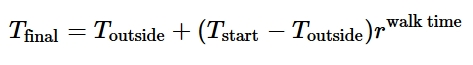The constant r is a number between 0 and 1, representing the heat exchange between the coffee cup and the outside environment. This constant depends on several factors, and may be hard to estimate without experimentation. We just chose it somewhat arbitrarily in this first example.

We will start by setting variables to represent the outside temperature and the rate of cooling and defining a function that computes the temperatures as the liquid cools. Then, type the lines of code representing the cooling law in a single code cell. Press Enter or click on Return to add new lines to the cell.

As discussed, we will first define the variables to hold the outside temperature and the rate of cooling:

```temp_out = 70.0
r = 0.9
```

After entering the preceding code on the cell, press Shift + Enter to execute the cell. Notice that after the cell is executed, a new cell is created.

### Note

Notice that we entered the value of `temp_out` as `70.0` even though the value is an integer in this case. This is not strictly necessary in this case, but it is considered good practice. Some code may behave differently, depending on whether it operates on integer or floating-point variables. For example, evaluating `20/8` in Python Version 2.7 results in `2`, which is the integer quotient of 20 divided by 8. On the other hand, `20.0/8.0` evaluates to the floating-point value `2.5`. By forcing the variable `temp_out` to be a floating-point value, we prevent this somewhat unexpected kind of behavior.

A second reason is to simply improve code clarity and readability. A reader of the notebook on seeing the value `70.0` will easily understand that the variable `temp_out` represents a real number. So, it becomes clear that a value of `70.8`, for example, would also be acceptable for the outside temperature.

Next, we define the function representing the cooling law:

```def cooling_law(temp_start, walk_time):
return temp_out + (temp_start - temp_out) * r ** walk_time
```

### Note

Please be careful with the way the lines are indented, since indentation is used by Python to define code blocks. Again, press Shift + Enter to execute the cell.

The `cooling_law()`function accepts the starting temperature and walking time as the input and returns the final temperature of the coffee. Notice that we are only defining the function, so no output is produced. In our examples, we will always choose meaningful names for variables. To be consistent, we use the conventions in the Google style of coding for Python as shown in http://google-styleguide.googlecode.com/svn/trunk/pyguide.html#Python_Language_Rules.

### Note

Notice that the exponentiation (power) operator in Python is `**` and not `^` as in other mathematical software. If you get the following error when trying to compute a power, it is likely that you meant to use the `**` operator:

`TypeError: unsupported operand type(s) for ^: 'float' and 'float'`

We can now compute the effect of cooling given any starting temperature and walking time. For example, to compute the temperature of the coffee after 10 minutes, assuming the initial temperature to be 185°F, run the following code in a cell:

```cooling_law(185.0, 10.0)
```

The corresponding output is:

```110.0980206115
```

What if we want to know what the final temperature for several walking times is? For example, suppose that we want to compute the final temperature every 5 minutes up to 20 minutes. This is where `NumPy` makes things easy:

```times = arange(0.,21.,5.)
temperatures = cooling_law(185., times)
temperatures
```

We start by defining `times` to be a `NumPy` array, using the `arange()` function. This function takes three arguments: the starting value of the range, the ending value of the range, and the increment.

### Note

You may be wondering why the ending value of the range is `21` and not `20`. It's a common convention in Computer Science, followed by Python. When a range is specified, the right endpoint never belongs to the range. So, if we had specified `20` as the right endpoint, the range would only contain the values `0`, `5`, `10`, and `15`.

After defining the `times` array, we can simply call the `cooling_law()` function with `times` as the second argument. This computes the temperatures at the given times.

### Note

You may have noticed that there is something strange going on here. The first time the `cooling_law()` function was called, the second argument was a floating-point number. The second time, it was a `NumPy` array. This is possible thanks to Python's dynamic typing and polymorphism. `NumPy` redefines the arithmetic operators to work with arrays in a smart way. So, we do not need to define a new function especially for this case.

Once we have the temperatures, we can display them in a graph. To display the graph, execute the following command line in a cell:

```plot(times, temperatures, 'o')
```

The preceding command line produces the following plot: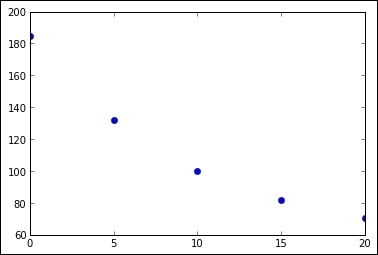The `plot()` function is a part of the `matplotlib` package, which will be studied in detail in Chapter 3, Graphics with matplotlib. In this example, the first two arguments to `plot()` are `NumPy` arrays that specify the data for the horizontal and vertical axes, respectively. The third argument specifies the plot symbol to be a filled circle.

We are now ready to tackle the original problem: should we mix the cream in at the coffee shop or wait until we get back to the office? When we mix the cream, there is a sudden drop in temperature. The temperature of the mixture is the average of the temperature of the two liquids, weighted by volume. The following code defines a function to compute the resulting temperature in a mix:

```def temp_mixture(t1, v1, t2, v2):
return (t1 * v1 + t2 * v2) / (v1 + v2)
```

The arguments in the function are the temperature and volume of each liquid. Using this function, we can now compute the temperature evolution when the cream is added at the coffee shop:

```temp_coffee = 185.
temp_cream = 40.
vol_coffee = 8.
vol_cream = 1.
initial_temp_mix_at_shop = temp_mixture(temp_coffee, vol_coffee, temp_cream, vol_cream)
temperatures_mix_at_shop = cooling_law(initial_temp_mix_at_shop, times)
temperatures_mix_at_shop
```

### Tip

Notice that we repeat the variable `temperatures_mix_at_shop` at the end of the cell. This is not a typo. The IPython notebook, by default, assumes that the output of a cell is the last expression computed in the cell. It is a common idiom to list the variables one wants to have displayed, at the end of the cell. We will later see how to display fancier, nicely formatted output.

As usual, type all the commands in a single code cell and then press Shift + Enter to run the whole cell. We first set the initial temperatures and volumes for the coffee and the cream. Then, we call the `temp_mixture()` function to calculate the initial temperature of the mixture. Finally, we use the `cooling_law()` function to compute the temperatures for different walking times, storing the result in the `temperatures_mix_at_shop` variable. The preceding command lines produce the following output:

```array([ 168.88888889,  128.3929    ,  104.48042352,   90.36034528,
82.02258029])
```

Remember that the `times` array specifies times from `0` to `20` with intervals of 5 minutes. So, the preceding output gives the temperatures at these times, assuming that the cream was mixed in the shop.

To compute the temperatures when considering that the cream is mixed after walking back to our office, execute the following commands in the cell:

```temperatures_unmixed_coffee = cooling_law(temp_coffee, times)
temperatures_mix_at_office = temp_mixture(temperatures_unmixed_coffee, vol_coffee, temp_cream, vol_cream)
temperatures_mix_at_office
```

We again use the `cooling_law()` function, but using the initial coffee temperature `temp_coffee` (without mixing the cream) as the first input variable. We store the results in the `temperatures_unmixed_coffee` variable.

To compute the effect of mixing the cream in after walking, we call the `temp_mixture()` function. Notice that the main difference in the two computations is the order in which the functions `cooling_law()` and `temp_mixture()` are called. The preceding command lines produce the following output:

```array([ 168.88888889,  127.02786667,  102.30935165,   87.71331573,
79.09450247])
```

Let's now plot the two temperature arrays. Execute the following command lines in a single cell:

```plot(times, temperatures_mix_at_shop, 'o')
plot(times, temperatures_mix_at_office, 'D', color='r')
```

The first `plot()` function call is the same as before. The second is similar, but we want the plotting symbol to be a filled diamond, indicated by the argument `'D'`. The `color='r'` option makes the markings red. This produces the following plot: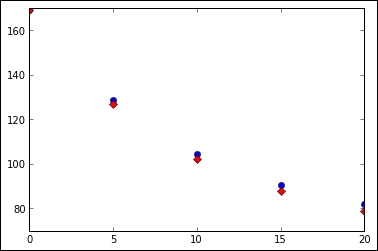Notice that, by default, all graphs created in a single code cell will be drawn on the same set of axes. As a conclusion, we can see that, for the data parameters used in this example, mixing the cream at the coffee shop is always better no matter what the walking time is. The reader should feel free to change the parameters and observe what happens in different situations.

Scientific plots should make clear what is being represented, the variables being plotted, as well as the units being used. This can be nicely handled by adding annotations to the plot. It is fairly easy to add annotations in `matplotlib`, as shown in the following code:

```plot(times, temperatures_mix_at_shop, 'o')
plot(times, temperatures_mix_at_office, 'D', color='r')
title('Coffee temperatures for different walking times')
xlabel('Waking time (min)')
ylabel('Temperature (F)')
legend(['Mix at shop', 'Mix at office'])
```

After plotting the arrays again, we call the appropriate functions to add the title (`title()`), horizontal axis label (`xlabel()`), vertical axis label (`ylabel()`), and legend (`legend()`). The arguments to all this functions are strings or a list of strings as in the case of `legend()`. The following graph is what we get as an output for the preceding command lines: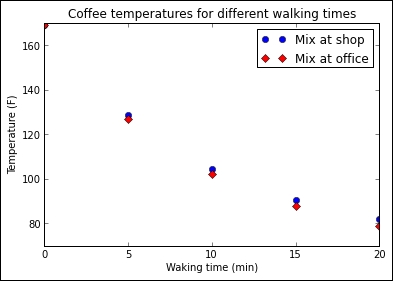There is something unsatisfactory about the way we conducted this analysis; our office, supposedly, is at a fixed distance from the coffee shop. The main factor in the situation is the outside temperature. Should we use different strategies during summer and winter? In order to investigate this, we start by defining a function that accepts as input both the cream temperature and outside temperature. The return value of the function is the difference of final temperatures when we get back to the office.

The function is defined as follows:

```temp_coffee = 185.
vol_coffee = 8.
vol_cream = 1.
walk_time = 10.0
r = 0.9
def temperature_difference(temp_cream, temp_out):
temp_start = temp_mixture(temp_coffee,vol_coffee,temp_cream,vol_cream)
temp_mix_at_shop = temp_out + (temp_start - temp_out) * r ** walk_time
temp_start = temp_coffee
temp_unmixed =  temp_out + (temp_start - temp_out) * r ** walk_time
temp_mix_at_office = temp_mixture(temp_unmixed, vol_coffee, temp_cream, vol_cream)
return temp_mix_at_shop - temp_mix_at_office
```

In the preceding function, we first set the values of the variables that will be considered constant in the analysis, that is, the temperature of the coffee, the volumes of coffee and cream, the walking time, and the rate of cooling. Then, we defined the `temperature_difference` function using the same formulas we discussed previously. We can now use this function to compute a matrix with the temperature differences for several different values of the cream temperature and outside temperature:

```cream_temperatures = arange(40.,51.,1.)
outside_temperatures = arange(35.,60.,1.)
cream_values, outside_values = meshgrid(cream_temperatures, outside_temperatures)
temperature_differences = temperature_difference(cream_values, outside_values)
```

The first two lines in the cell use the `arange()` function to define arrays that contain equally spaced values for the cream temperatures and outside temperatures. We then call the convenience function, `meshgrid()`. This function returns two arrays that are convenient to calculate data for three-dimensional graphs and are stored in the variables `cream_values` and `outside_values`. Finally, we call the `temperature_difference()` function, and store the return value in the `temperature_differences` array. This will be a two-dimensional array (that is, a matrix).

We are now ready to create a three dimensional plot of the temperature differences:

```from mpl_toolkits.mplot3d import Axes3D
fig = figure()
fig.set_size_inches(7,5)
ax.plot_surface(cream_values, outside_values, temperature_differences, rstride=1, cstride=1, cmap=cm.coolwarm, linewidth=0)
xlabel('Cream temperature')
ylabel('Outside temperature')
title('Temperature difference')
```

In the preceding code segment, we started by importing the `Axes3D` class using the following line:

```from mpl_toolkits.mplot3d import Axes3D
```

This class, located in the `mpl_toolkits.mplot3d` module, is not automatically loaded. So, it must be explicitly imported.

Then we create an object `fig` of the class `figure`, set its size, and generate an object `ax` that is an object of the class `Axes3D`. Finally, we call the `ax.plot_surface()` method to generate the plot. The last three command lines set the axis labels and the title.

### Note

In this explanation, we used some terms that are common in object-oriented programming. A Python object is simply a data structure that can be handled in some specialized way. Every object is an instance of a class that defines the object's data. The class also defines methods, which are functions specialized to work with objects belonging to the class.

The output of the preceding command lines will produce the following graph: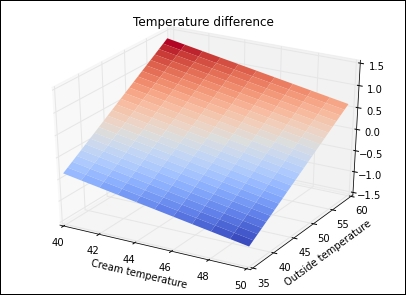Notice the `cmap=cm.coolwarm` argument in the call to `ax.plot_surface()`. This sets the color map of the plot to `cm.coolwarm`. This color map conveniently uses a blue-red gradient for the function values. As a result, negative temperature differences are shown in blue and positive temperatures in red. Notice that there seems to be a straight line that defines where the temperature difference transitions from negative to positive. This actually corresponds to values where the outside temperature and the cream temperature are equal. It turns out that if the cream temperature is lower than the outside temperature, we should mix the cream into the coffee at the coffee shop. Otherwise, the cream should be poured in the office.